# S14E2 - Acid Strength and Acid-Base Conjugate Pairs

## Another Bronsted-Lowry Acid-Base Reaction.

ex:  Let's look at the reaction between ammonia (a base) and hydrochloric acid: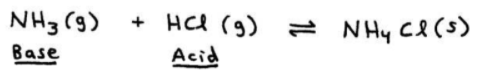As you can see in the second image below, the lone pair on NH3 actively receives the H+ (proton) from the Bronsted-Lowry acid.

So while we say that the acid is the proton-donor, it's the Bronsted-Lowry base (NH3) that actively "goes out and grabs" the proton (H+).

The result is an H+ transfter that generates the products - the conjugate base of our original acid, and the conjugate acid of our original base:=====

## Acid Dissociation Reaction Practice Problems.

ex:   HC2H3O2

HC2H3O2 (aq)   ⇌   H+ (aq)   +   C2H3O2 - (aq)

- or, handwritten so it's easy to see: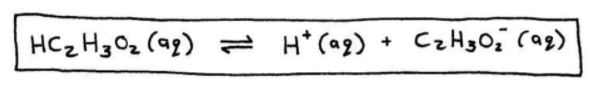-----

ex:   H2SO4

H2SO4 (aq)   ⇌   H+ (aq)   +  HSO4 - (aq)

- or, handwritten so it's easy to see:-----

ex:   [Al(H2O)6]3+

Al(H2O)63+ (aq)   ⇌   H+ (aq)   +  Al(H2O)5(OH)2+ (aq)

- or, handwritten so it's easy to see: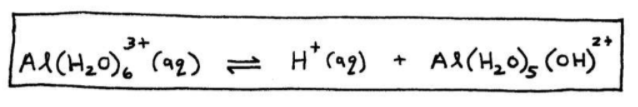=====

## Acid Strength.

➞ consider the reaction of the monoatomic acid, HA, with water:

HA (aq)   +   H2O (l)   ⇌   H3O+ (aq)   +   A- (aq)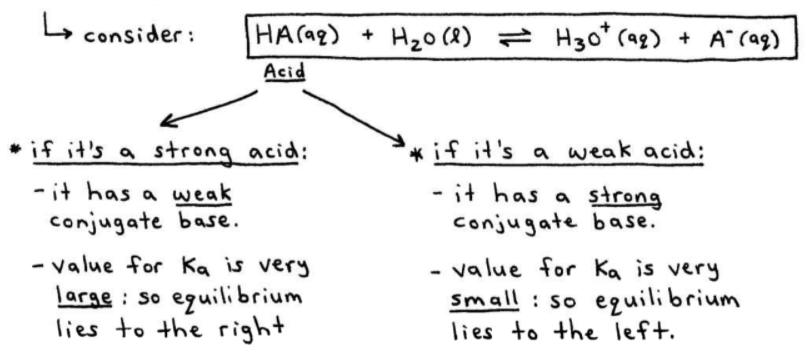As stated already in the image above,

If HA is a strong acid, then:
- it has a weak conjugate base.
- the value for Ka is very large: so equilibrium lies to the right.

If HA is a weak acid, then:
- it has a strong conjugate base.
- the value for Ka is very small: so equilibrium lies to the left.

-----

NOTE - The strongest acid and strongest base always end up on the same side of a reaction, and equilibrium lies away from them.

So in the above general reaction, if HA is a stronger acid than H3O+, then H2O must be a stronger base than A-

=====

## Table of Ka Values for Some Common Monoprotic Acids.

monoprotic = acid has just one acidic proton (H+).

In Table 14-1 below, the strong acids HCl, HNO3, HClO4, H2SO4 are not listed because their "Ka values" are so high...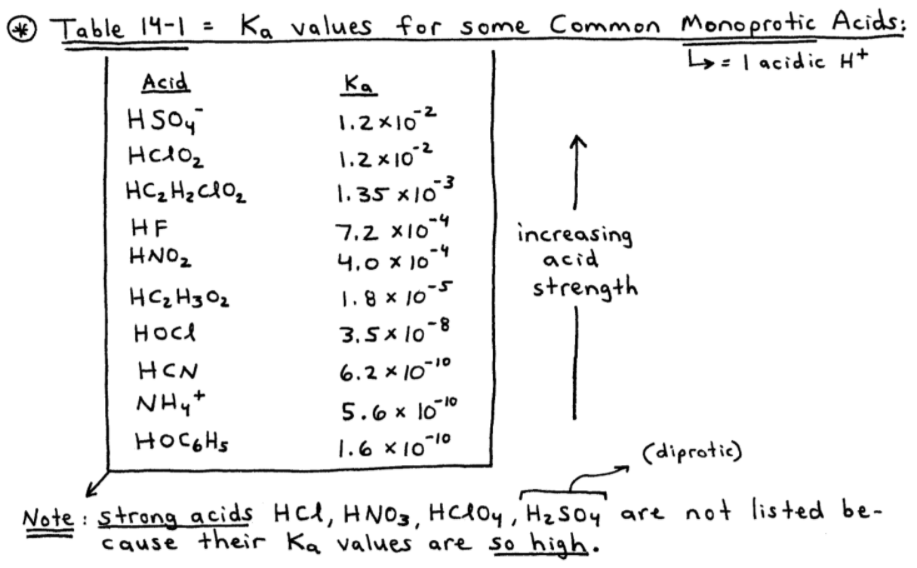Here's an acid-strength practice problem where Table 14-1 (above) will come in handy.

ex:  Using Table 14-1, arrange the following bases from strongest to weakest base.

F-   ,   Cl-   ,   NO2-   ,   CN-

To do this problem, we have to remember:

➞ the "weakest base" is the conjugate of the "strongest acid."
➞ the "strongest base" is the conjugate of the "weakest acid."So, we have:

CN-   >   NO2-   >   F-   >   Cl-

=====

Stick around for the 3rd installment of SECTION 14 - Acids and Bases,

where we'll talk about the pH scale and pH calculations...